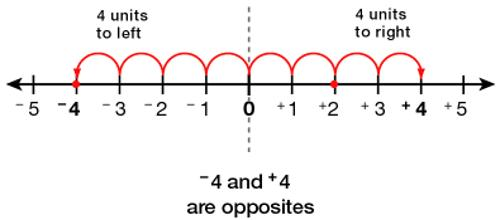Ordering Numbers

Ordering Numbers are numbers associated with a purchase or merchandise order. Numbers have an order or arrangement. The number two is between one and three. Three or more numbers can be placed in order. A number may come before the other numbers or it may come between them or after them.

Example: If we start with the numbers 4 and 8, the number 5 would come between them, the number 9 would come after them and the number 2 would come before both of them.

The order may be ascending (getting larger in value) or descending (becoming smaller in value).

How to Compare Two Numbers?

When we compare two numbers, there are three possibilities:

• The first number is greater than the second (4 > 2).
• The second number is greater than the first (2 < 3)
• The two numbers are equal (6 = 6)How to Order Numbers?

Numbers have an order or arrangement. They can be  arrange  from least to greatest or from greatest to least. When ordering numbers, we can compare the numbers two at a time. We can also use the following method.

Example:

Arrange the following numbers in increasing order: 425 876, 425 987, 425 856.

If we start with the numbers 10 and 12, the number 11 would come between them, the number 13 would come after them and the number 9 would come before both of them.

The standard ordering among real numbers agrees with the standard ordering among natural numbers, i.e. nonnegative integers. This takes some work to prove rigorously, but the sociological proof is simple: Any sane mathematician would refuse to accept as standard any ordering among real numbers that didn’t agree with the standard ordering among natural numbers.

Information Source: